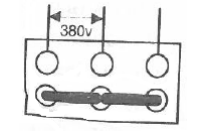# Induction motor

EXERCISE : STUDY OF AN INDUCTION MOTOR IN A RADIO TELESCOPE

Figure 1 shows the overall infrastructure of a radio telescope which may be considered as a measuring instrument. Figure 2 shows its simplified kinematic diagram.
The rotational movement of the platform (R1) with respect to a fixed frame (Bâti S0) is provided by an asynchronous motor (M1) controlled in both directions of rotation. Two tests were performed on this motor:
– No-load test (“à vide”) : U=380V between phases, 50 Hz, I0 = 3A, P0 = 500W,
– Rated load test (“à charge nominale”): U=380 V between phases, 50Hz, I = 10A , Pa = 5kW, n=720 rpm (tr/min).
The resistance of a stator winding is R = 0.5 Ω1- The following coupling is performed on the terminal plate :What type of coupling is? Give the voltages indicated on motor descriptive plate (“sur la plaque signalétique”).
2- Determine the stator iron losses Pfs and mechanical losses Pmec knowing that : Pfs =( 3/2)Pmec

3- Calculate the power cosφ0 factor at no-load and deduce the reactive power at no-load.

4- For operation at rated load, calculate :
a) Stator joule losses Pjs .           b) Transmitted power Ptr.        c) Slip g.            d) Rotor joule losses Pjr.
e) Useful output power Pu.         f) Efficiency η .        g) Power factor cosφ.        h) Impedance of each stator winding Z.
5- When the load torque applied to M1 is Tr = 40 Nm, is it (motor) capable of radio telescope rotational movement? Justify your answer.

CORRECTION